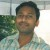# Sample Size Calculation

Six Sigma – iSixSigma Forums General Forums Tools & Templates Sample Size Calculation

Viewing 2 posts - 1 through 2 (of 2 total)
• Author
Posts
• #54358Jon
Guest

I’m a master student in public health and is doing a research. i’m trying to correct my sample size calculation. My study is related to Thalassaemia disease, therefore i actually use the probability of death due to cardiac complication in chelated and non-chelated thalassaemia patients. i calculated my sample using PS software based on the proportion of cardiac disease but i wanted to recheck by the formula using E, margin of error. (In which i’m not very sure if) the E in my study is the difference between the prevalence rate of the population and sample referring to Thalassaemia prevalence rate or the Cardiac disease. I’ve read a book that an example they set E at 20% of Population prevalence prevalence rate.

Thank you

0
#195047Prabhu V
Participant

Hi,

From your query the population prevalence rate of 20% can be the proportion on the discrete sample size formula = (Z/E)^2*p(1-p), where p is proportion.
E is the margin of error described as the level of precision desired from the sample in units of proportion (generally 5% or 1% on critical situations).

If my above opinion were correct, on the above situation the sample size requirement would be 246 samples on 95% CI and 5% error. (6146 samples on 95% CI and 1% error)

Kindly confirm do you agree on it.

Thanks.

0
Viewing 2 posts - 1 through 2 (of 2 total)

You must be logged in to reply to this topic.